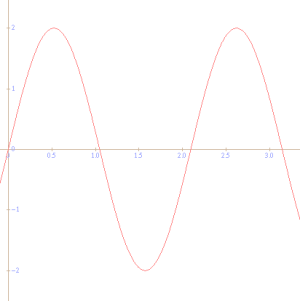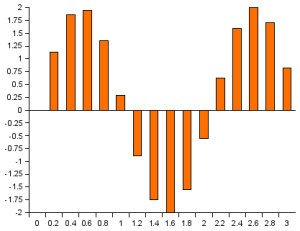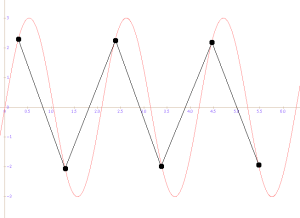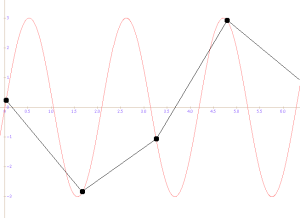## VoIP Basics: Converting Voice to Digital Form

Are you interested in Voice over IP? Would you like to know more about its background? This text begins a series that should shed some light on it.

Let's start with the beginning. VoIP sends digitized voice across computer networks. So how do we convert voice to the digital form?

When converting an analog signal (be it speech or another noise), you need to consider two important factors: sampling and quantization. Together, they determine the quality of the digitized sound.

• Sampling is about the sampling rate — i.e. how many samples per second you use to encode the sound.
• Quantization is about how many bits you use to represent each sample. The number of bits determines the number of different values you can represent with each sample.

Figures 1 and 2 show the idea of sampling — Figure 1 is the original analog signal, while Figure 2 shows the digitized form as a sequence of discrete samples.### Quantization

As mentioned above, quantization is about how many bits you use to represent individual sound samples. In practice, we want to work with whole bytes, so let's consider 8 or 16 bits.

With 8-bit samples, each sample can represent 256 different values, so we can work with whole numbers between -128 and +127. Because of the whole numbers, it is inevitable that we introduce some noise into the signal as we convert it to digital samples. For example, if the exact analog value is "7.44125", we will represent it as "7". As we do this with each sample in the sequence, we slightly distort the signal — inject noise, in other words.

It turns out 8-bit samples do not result in a good quality. With only 256 sample values, the analog-to-digital conversion adds too much noise. The situation improves a lot if we switch to 16-bit samples as 16 bits give us 65536 different representations (from -32768 to +32767). 16-bit samples are what you will find on a CD and what VoIP codecs use as their input.

### Sampling

Now that we have decided what sample size to use (16 bits), let's look at sampling rates. The table below shows three frequently used sampling rates:

Type Transmitted Bandwidth Sampling Frequency
Telephone Speech 300-3400 Hz 8 kHz
Wide Band Speech 50-7000 Hz 16 kHz
CD quality audio 20-20000 Hz 44.1 kHz

With VoIP, you will most frequently encounter the sampling rate of 8 kilohertz. The frequency of 16 kHz can be used now and then in situations when a higher quality audio is required (with proportionally higher Internet bandwidth consumption).

The choice of sampling frequencies for the individual types of audio is not random. There is a rule (based on the work of Nyquist and Shanon) that the sampling frequency needs to be equal or greater than two times the transmitted bandwidth. Figures 3 and 4 show why this is required.In Figure 3, the sinusoid represents the original analog sound. The large black dots are where we read our samples. Note that we take two samples in each period, i.e. the sampling rate is two times the frequency of the sound. This is the absolute minimum that will allow us to reconstruct a signal that is still comprehensible. It certainly won't be a hi-fi sound but it will have the correct frequency - see the thin black lines in the picture.The Figure 4 shows a situation where we take less than two samples per period. The thin black lines show what would happen after we feed the samples into a digital-to-analog converter — we would hear something different from the original, a sound with lower frequency. This problem is known as "aliasing" since the lower frequency appears to be an "alias" to the original correct one.

### Summary

In this piece, we discussed a conversion of voice to a digital format. We considered the influence of sampling frequency and of the sample's size. It's good to remember that VoIP most frequently works with the sampling frequency of 8 kilohertz and each sample is stored in 16 bits.

Next section: Overview of Audio Codecs